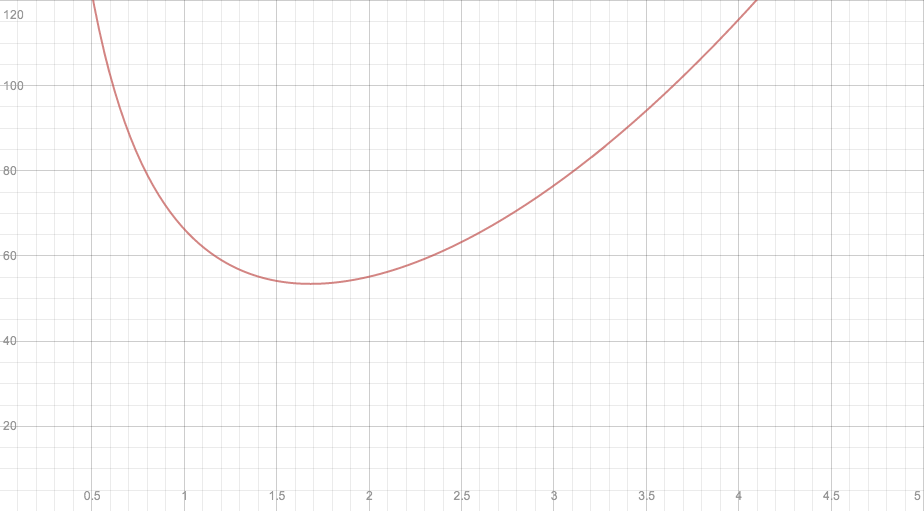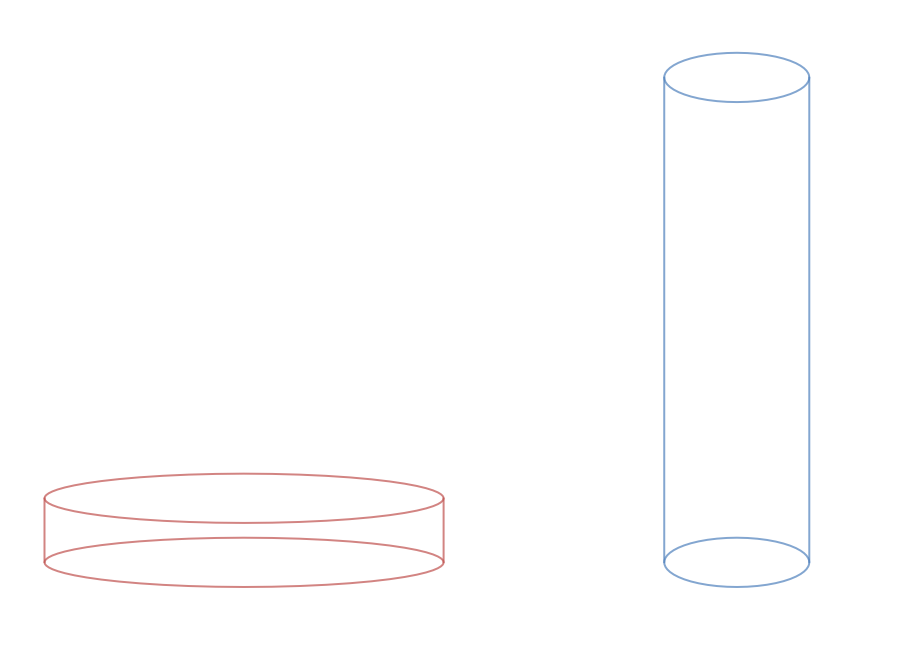## Canalysis

No pantry is complete without an ample supply of canned goods.  In addition to the variety of food that exists in can form, canned foods tend to be less expensive and have a longer shelf life than their uncanned counterparts.  It's no surprise, then, that canned food sales go up when the economy goes down.  But are we really saving as much as we could?  Or are canned food companies passing on unnecessary cost?

When you go to the supermarket, you've probably noticed that soup cans tend to be taller than they are wide.  The opposite is true for other products, like canned tuna. Still other cans have a shape somewhere in between.  Let's try to find out if one of these shapes is better than the others!One way to determine a "best" shape is to find the one that stores the most food using the least amount of material.  If manufacturers are able to minimize the amount of material used, it may result in a cheaper product.  So, let's say we have a fixed volume of food that we want to store in a cylindrical can using the smallest amount of material.  In other words, we want to make the surface area small.  To do this, we need to understand the relationship between volume and surface area.

You may remember that a cylinder with radius r and height h has volume (V) and surface area (S) given by the formulas

V = πr2h,

S = 2πr2 + 2πrh.

(For some discussion on where these formulas come from, see the comments below.)

If the volume is constant, then we can solve for the height in terms of it: hVr2. In turn, we get S = 2πr2 + 2V/r.  In other words, the surface area is a function of the radius!

This function is a little hard to wrangle, though, especially without Calculus.  The problem is that if you want a small surface area, the radius can't be too big because 2πr2 is large when r is large.  But it also can't be too small, because 2V/r is large when r is small.

Thankfully, there's a value of r which is just right - you can see it from the graph below when V = 30.  (Click through to explore how S changes as V changes!)It turns out that regardless of the volume, the surface area is smallest when the can's diameter equals its height.  Of the cans up above, though, only one has this property.  The others could hold the same amount using less material.  So why don't they?

Well, this isn't necessarily a manufacturing failure; it may be a failure of our model. For example, the top of the can is often the last piece attached, and sometimes is even manufactured in a different location.  Consequently, it may cost a different amount.  If the top is c times as expensive per square inch as the rest of the can, then as c increases, the cheapest design has a smaller and smaller radius.  This makes intuitive sense - if the top is expensive, we should make it smaller.  (The orange graph represents the cost if the top were twice as expensive as the rest of the can, assuming the other materials cost \$1/in.2; as before, you can click through to explore what happens when c and V vary.)Of course, this is just one possible factor affecting a can's shape.  There are undoubtedly others.  For example, one factor may be psychological: taller, thinner cans may appear larger than shorter, rounder cans.  Would you believe that the blue and red cylinders actually have the same volume?  (Click through to play around with these cylinders; you can adjust the radii and heights, but their volumes will remain identical.)There are undoubtedly other possible factors as well.  But you folks are smart - I'm sure you can come up with alternative explanations on your own!

Teachers: interested in discussing other explanations with your students?  Then check out our new lesson, Canalysis!

## 7 thoughts on “Canalysis”

1. >V = πr2h, S = 2πr2 + 2πrh.

Students think of these as black boxes they need to memorize. Can you add in something about how they could build these formulas themselves?

1. Matt says:

Hi Sue,

Great point, and I completely agree. I thought about including some discussion of where these formulas come from, but ultimately decided against it for sake of the post’s flow. However, I’m happy to talk about it a bit in the comments here.

Let’s start with the surface area formula. In my mind, the best way to think about it is to view the cylinder in three parts: the top, the bottom, and the curved side. Since the top and bottom are both circles, each one has area πr2. Next, since there are two circles, we get two copies of this area, which is where the 2πr2 term comes from.

For the side, I always imagine taking a pair of scissors and cutting a line from top to bottom of the cylinder and unrolling the side (you could do this to an empty paper towel roll, for example). The result is that you’ll get a rectangle, whose height is equal to the height of the cylinder, and whose length is equal to the circumference of the circle at either end of the cylinder. But since the circle has radius r, it has circumference 2πr, so the length of the rectangle is 2πr. Since the area of a rectangle is simply the length times the height, we get that the area of the side of the cylinder is 2πrh, and this accounts for the second term in the surface area formula.

As for the volume, the key point is that for a cylinder, as for prisms, the volume is given by the base area times the height (this follows, for example, from Cavalieri’s principle). In the case of a cylinder, since the base is a circle, the base area is πr2, and the height is h. Therefore the volume is the product of these two quantities, or πr2h.

Of course, I’m sure none of this is news to you. But if any other readers have forgotten where these formulas come from, hopefully this will be of some use. I’ve also updated the post to reflect this discussion.

Thanks for the feedback!

2. Hi. Admittedly unrelated to optimum can sizes, but related to cans, is Canstruction (http://canstruction.org) . This is a combination food charity and architectural competition involving giant structures made entirely of cans which ultimately go to local food banks. Events are held around the world, including one from Feb 1 - 11, 2013 at the World Financial Center in New York City. If an event is held near you, you should take a look - you would be amazed at the detail and beauty of some of these structures.

Jerry

1. Matt says:

Hey Jerry,

Those structures are pretty cool. Thanks for sharing!

3. jachmens says:

Hi Matt, i am doing a similar investigation to this activity but i am confused on how to graph the function from Act one (2). I tried doing this quadratically by hand but i am not sure of the values to insert into the equation SA = 2πr^2 x 16π/r. Can you please be of help on how to plot the surface area so that it is a function of only the radius. Thank you.

1. Matt says:

Hi there,

Graphing the function by hand is going to be difficult at the Pre-Calculus level (indeed, the lesson we provide suggests using technology, like the graphing calculator at Desmos.com, to see what the graph of the function looks like). The expression you have given for the surface area is correct (though I'm assuming you meant 2πr^2 + 16π/r, not 2πr^2 x 16π/r), and this is a function of only the radius. It's just not a quadratic function; it's a rational one!

Hope this helps. Let me know if you have more questions.